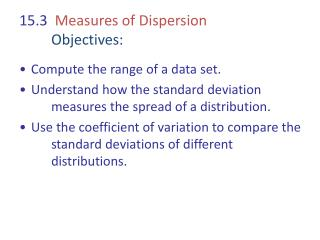# 15.3 Measures of Dispersion Objectives: - PowerPoint PPT PresentationDownload Presentation15.3 Measures of Dispersion Objectives:

15.3 Measures of Dispersion Objectives:
Download Presentation## 15.3 Measures of Dispersion Objectives:

- - - - - - - - - - - - - - - - - - - - - - - - - - - E N D - - - - - - - - - - - - - - - - - - - - - - - - - - -
##### Presentation Transcript

1. 15.3 Measures of DispersionObjectives: • Compute the range of a data set. • Understand how the standard deviation measures the spread of a distribution. • Use the coefficient of variation to compare the standard deviations of different distributions.

2. The Range of a Data Set Example: Find the range of the heights of the people listed in the accompanying table. Solution:

3. Deviation from the Mean Example: Find the deviations from the mean for the data set { 16, 14, 12, 21, 22 }. Solution: The mean is = = 17. The deviations from this mean are: So what is the average deviation? __________

4. Standard Deviation For our previous example:

5. Standard Deviation cont. (2) So the variance is the square of the Standard Deviation.

6. Standard Deviation cont. (3) Example: A company has hired six interns. After 4 months, their work records show the following number of work days missed for each worker: 0, 2, 1, 4, 2, 3 Find the standard deviation of this data set. Solution: First we find the mean. Mean = Now we find the squares of the deviations of the data values from the mean. So the standard deviation is

7. Standard Deviation cont. (4) Example: The following are the closing prices for a stock for the past 20 trading sessions. 37, 39, 39, 40, 40, 38, 38, 39, 40, 41, 41, 39, 41, 42, 42, 44, 39, 40, 40, 41 What is the standard deviation for this data set? Solution: Mean = , (sum of the closing prices is 800) Cont. SD (5)

8. Standard Deviation cont. (5) We create a table with values that will facilitate computing the standard deviation. So the standard deviation is

9. Comparing Standard Deviations All three distributions have a mean and median of 5; however, as the spread of the distribution increases, so does the standard deviation.

10. Comparing Standard Deviations cont. (2) • Of course, it is not only the range of a data set that affects the standard deviation. Which of these data sets has a larger standard deviation? A = { 1, 8, 9 } , B = { 1, 5, 9 } sA = _______ sB = _______ • Rank the following data sets in order of increasing variability. C = { 1, 5, 5, 9 } ____ D = { 2, 4, 5, 9 } ____ E = { 1, 4, 6, 9 } ____ F = { 2, 2, 8, 8 } ____

11. The Coefficient of Variation The Coefficient of Variation gives us another measure by which to compare the variability of two data sets. It expresses the standard deviation as a percentage of the mean. Example: Relatively speaking there is more variation in the weights of the 1st graders than in the weights of the NFL players. 1stGraders Mean: 30 pounds SD: 3 pounds CV: NFL Players Mean: 300 pounds SD: 10 pounds CV:

12. The Coefficient of Variation (2) Example: Use the coefficient of variation to determine whether the women’s 100-meter race or the men’s marathon has had more consistent times over the five Olympics listed. Does “more consistent” mean that the CV will be larger or smaller ? _________

13. The Coefficient of Variation (3) Solution: Using the coefficient of variation as a measure, there is less variation (and so more consistency) in the times for the marathon than for the 100-meter race. 100 Meters Mean: 10.796 SD: 0.163 CV: Marathon Mean: 7,891.4 SD: 83.5 CV: